2022: SklogWiki celebrates 15 years on-line

# Building up a square lattice

• Consider:
1. a square simulation box whose sides are of length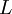$\left. L \right.$
2. a number of lattice positions,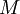$\left. M \right.$ given by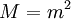$\left. M = m^{2} \right.$ ; with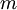$m$ being a positive integer
• The$\left. M \right.$ positions are those given by: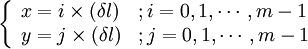$\left\{ \begin{array}{ll} x = i \times (\delta l) &; i=0,1,\cdots, m-1 \\ y = j \times (\delta l) &; j=0,1,\cdots, m-1 \\ \end{array} \right.$

where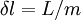$\left. \delta l = L/m \right.$

## Atomic position(s) on a square cell

• Number of atoms per cell: 1
• Coordinates:

Atom 1: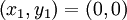$\left( x_1, y_1\right) = \left( 0, 0 \right)$